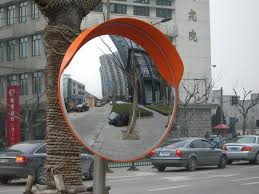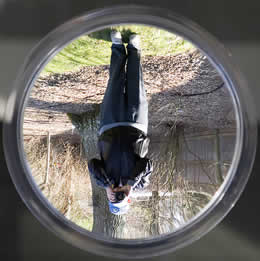# Difference between Convex and Concave Mirror

##### Key Difference: Concave and convex are two classes of spherical mirrors. A concave mirror is a spherical mirror in which the reflecting surface and the center of curvature fall on the same side of the mirror. On the other hand, a convex mirror is a spherical mirror in which the reflecting surface and the center of curvature lie on the opposite sides of the mirror.

Convex and concave are two types of spherical mirrors. A spherical mirror is actually a mirror whose surface forms a part of a hollow sphere. The sphere can be made from any type of polished metal surface like glass. The center of the mirror is called the pole and the radius through the pole is called the axis of the mirror.A convex mirror is a spherical mirror in which the reflecting surface and the center of curvature lie on the opposite sides of the mirror. In other words, if the mirror coating lies in the inside of the spherical surface, then it is known as a convex mirror. It is also known as a diverging mirror as – when a beam of light is directed on a convex mirror, the mirror allows the initial parallel rays to diverge after striking it. They are known to provide wider views, and therefore are primary used as automobile side mirrors.A concave mirror is a spherical mirror in which the reflecting surface and the center of curvature fall on the same side of the mirror. In other words, if the mirror coating lies outside of the spherical surface, then it is known as a concave mirror. This type of mirror forms a part of the simplest image forming optical system. Therefore, it has many applications. The image in a concave mirror is seen inverted. However, the image can be of the same size or magnified; depends upon the distance from the concave mirror.

Comparison between Convex and Concave Mirror:

 Concave Mirror Convex Mirror Structure A concave mirror is a spherical mirror in which the reflecting surface and the center of curvature fall on the same side of the mirror. In other words, if the mirror coating lies outside of the spherical surface, then it is known as a concave mirror. A convex mirror is a spherical mirror in which the reflecting surface and the center of curvature lie on the opposite sides of the mirror. In other words, if the mirror coating lies in the inside of the spherical surface, then it is known as a convex mirror. Other name Converging Mirror Diverging Mirror, Fish eye mirror Image projection Can be projected on a screen Cannot be projected on a screen. Meaning Concave means “hollowed or rounded”. Convex means “curved or rounded like the outside of a sphere or circle”. Image (C stands for center of curvature, F for focal point and V for vertex) Position of object (infinity) Position of Image (At F) Character of Image (Real, zero size) Position of object (between infinity and C) Position of Image (Between F and C) Character of Image (real, inverted, diminished)  Position of object (AT C) Character of Image (Real, inverted, same size) Position of object (Between C and F)  Position of Image (Between C and infinity). Character of Image (Real, inverted, magnified) Position of object (Between F and V) Position of Image (From –infinity to V) Character of Image (Virtual, upright, magnified) Position of object (AT V) Position of Image (At V) Character of Image (Virtual, upright, same size) Position of object (infinity) Position of Image (At F) Character of Image (Virtual, zero size) Position of object (between infinity and V) Position of Image (Between F and V) Character of Image (Virtual, upright, Diminished)  Position of object (AT V) Position of Image (At V) Character of Image (Virtual, upright, same size)

Thank u for this useful article.

Thanks it is useful for me.

Thx It was very helpful :-)

It is very use ful and try like this only ☺☺☺

Thank for uploading such a useful material i want differences on different topics.

Very useful thnx

Its fabulous

very useful

It was useful thanks